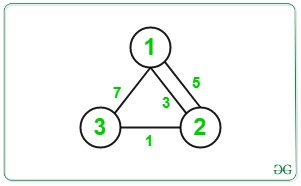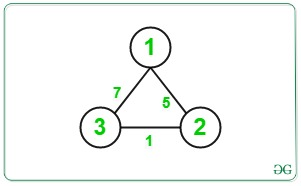# Minimum Cost using Dijkstra by Modifying Cost of an Edge

• Difficulty Level : Hard
• Last Updated : 30 Jun, 2022

Given an undirected weighted graph of N nodes and M edges in the form of a tuple lets say {X, Y, Z} such that there is an edge with cost Z between X and Y. We are supposed to compute the minimum cost of traversal from node 1 to N. However, we can perform one operation before the traversal such that we can reduce the cost of any edge lets say, C to C / 2 (integer division)

Examples:

Input: N = 3, M = 4, Edges = {{1, 2, 3}, {2, 3, 1}, {1, 3, 7}, {2, 1, 5}}
Output:
Explanation:Minimum Cost from source node 1 to destination node N is = 3/2 + 1 = 1 + 1 = 2.
Input: N = 3, M = 3, Edges = {{2, 3, 1}, {1, 3, 7}, {2, 1, 5}}
Output:
Explanation:Minimum Cost from source node 1 to destination node N is = 7/2 = 3.

Approach: The idea is to consider every edge to be modified and try to minimize the overall cost by reducing its cost. The main idea is to break the path between node 1 to N into the path from 1 to any vertex u i.e., path(1 to u) and from node N to any vertex v i.e., path(n to v) for all u and v such that u to v forms an edge. We can easily compute the distance from any node lets say, source to all other nodes in the graph by applying single source shortest path algorithm, Dijkstra Algorithm. In this problem we would be applying the Dijkstra Algorithm twice by choosing sources as 1 and N separately and storing the cost to reach each node in the array dist_from_source[] and dist_from_dest[] respectively.
After we have computed these two arrays we can easily compute the cost associated after modifying each edge. Lets consider an edge u to v and let the cost associated with it be c. If we try to modify this edge we can compute the minimum cost from 1 to N as dist_from_source[u] + dist_from_dest[v] + c / 2. Doing this for all the edges and minimizing it we can get the minimum cost to travel from source 1 to destination N

1. Perform a Dijkstra Algorithm to find the single source shortest path for all the vertex from node 1 and store it in an array as dist_from_source[].
2. Perform a Dijkstra Algorithm to find the single source shortest path for all the vertex from node N and store it in an array as dist_from_dest[].
3. Declare a variable minCost and assign it to a very large number initially.
4. Traverse all the given edges [u, v, c] and reduce it like formula discussed above and update the minCost variable as:

minCost = min(minCost, dist_from_source[u] + c/2 + dist_from_dest[v])
where,
c is the cost of current edge,
dist_from_source[u] is cost of path from node 1 to u
dist_from_source[v] is cost of path from node N to v

1. Repeat this process for all the edges and correspondingly update the minCost variable.

2. Print the value of minCost after the above step.

Below is the implementation of the above approach:

## C++14

 `// C++ program for the above approach` `#include ` `using` `namespace` `std;` `#define INF 1e9`   `// Function for Dijkstra Algorithm to` `// find single source shortest path` `void` `dijkstra(``int` `source, ``int` `n,` `              ``vector >` `                  ``adj[],` `              ``vector<``int``>& dist)` `{` `    ``// Resize dist[] to N and assign` `    ``// any large value to it` `    ``dist.resize(n, INF);`   `    ``// Initialise distance of source` `    ``// node as 0` `    ``dist = 0;`   `    ``// Using min-heap priority_queue` `    ``// for sorting wrt edges_cost` `    ``priority_queue,` `                   ``vector >,` `                   ``greater > >` `        ``pq;`   `    ``// Push the current dist` `    ``// and source to pq` `    ``pq.push({ dist, source });`   `    ``// Until priority queue is empty` `    ``while` `(!pq.empty()) {`   `        ``// Store the cost of linked` `        ``// node to edges` `        ``int` `u = pq.top().second;` `        ``// int d = pq.top().first;`   `        ``// Pop the top node` `        ``pq.pop();`   `        ``// Iterate over edges` `        ``for` `(``auto``& edge : adj[u]) {`   `            ``// Find the starting and` `            ``// ending vertex of edge` `            ``int` `v = edge.first;` `            ``int` `w = edge.second;`   `            ``// Update the distance of` `            ``// node v to minimum of` `            ``// dist[u] + w if it is` `            ``// minimum` `            ``if` `(dist[u] + w < dist[v]) {` `                ``dist[v] = dist[u] + w;` `                ``pq.push({ dist[v], v });` `            ``}` `        ``}` `    ``}` `}`   `// Function to find the minimum cost` `// between node 1 to node n` `void` `minCostPath(` `    ``vector > >& edges,` `    ``int` `n, ``int` `M)` `{`   `    ``// To create Adjacency List` `    ``vector > adj;`   `    ``// Iterate over edges` `    ``for` `(``int` `i = 0; i < M; i++) {`   `        ``// Get source, destination and` `        ``// edges of edges[i]` `        ``int` `x = edges[i].first;` `        ``int` `y = edges[i].second.first;` `        ``int` `z = edges[i].second.second;`   `        ``// Create Adjacency List` `        ``adj[x].push_back({ y, z });` `        ``adj[y].push_back({ x, z });` `    ``}`   `    ``// To store the cost from node 1` `    ``// and node N` `    ``vector<``int``> dist_from_source;` `    ``vector<``int``> dist_from_dest;`   `    ``// Find the cost of travel between` `    ``// source(1) to any vertex` `    ``dijkstra(1, n + 1, adj, dist_from_source);`   `    ``// Find the cost of travel between` `    ``// destination(n) to any vertex` `    ``dijkstra(n, n + 1, adj, dist_from_dest);`   `    ``// Initialise the minimum cost` `    ``int` `min_cost = dist_from_source[n];`   `    ``// Traverse the edges` `    ``for` `(``auto``& it : edges) {`   `        ``// Get the edges` `        ``int` `u = it.first;` `        ``int` `v = it.second.first;` `        ``int` `c = it.second.second;`   `        ``// Find the current cost from` `        ``// node 1 to u and node u to v` `        ``// and node v to N with only` `        ``// current edge cost reduced` `        ``// to half` `        ``int` `cur_cost = dist_from_source[u]` `                       ``+ c / 2` `                       ``+ dist_from_dest[v];`   `        ``// Update the min_cost` `        ``min_cost = min(min_cost, cur_cost);` `    ``}`   `    ``// Print the minimum cost` `    ``cout << min_cost << ``'\n'``;` `}`   `// Driver Code` `int` `main()` `{` `    ``// Give Nodes and Edges` `    ``int` `N = 3;` `    ``int` `M = 3;`   `    ``// Given Edges with cost` `    ``vector > > edges;`   `    ``edges.push_back({ 2, { 3, 1 } });` `    ``edges.push_back({ 1, { 3, 7 } });` `    ``edges.push_back({ 2, { 1, 5 } });`   `    ``// Function Call` `    ``minCostPath(edges, N, M);` `    ``return` `0;` `}`

## Javascript

 ``

## Python3

 `# Python3 program for the above approach` `import` `heapq as hq`   `INF ``=` `1e9`   `# Function for Dijkstra Algorithm to` `# find single source shortest path` `def` `dijkstra(source, n, adj, dist):` `    ``# Initialise distance of source` `    ``# node as 0` `    ``dist ``=` `0`   `    ``# Using min-heap priority_queue` `    ``# for sorting wrt edges_cost` `    ``pq ``=` `[]`   `    ``# Push the current dist` `    ``# and source to pq` `    ``hq.heappush(pq, (dist, source))`   `    ``# Until priority queue is empty` `    ``while` `pq:`   `        ``# Store the cost of linked` `        ``# node to edges` `        ``d, u ``=` `hq.heappop(pq)`   `        ``# Iterate over edges` `        ``for` `v,w ``in` `adj[u]:`   `            ``# Update the distance of` `            ``# node v to minimum of` `            ``# dist[u] + w if it is` `            ``# minimum` `            ``if` `dist[u] ``+` `w < dist[v]:` `                ``dist[v] ``=` `dist[u] ``+` `w` `                ``hq.heappush(pq, (dist[v], v))`     `# Function to find the minimum cost` `# between node 1 to node n` `def` `minCostPath(edges, n, M):`   `    ``# To create Adjacency List` `    ``adj ``=` `[[]``for` `_ ``in` `range``(``100005``)]`   `    ``# Iterate over edges` `    ``for` `i ``in` `range``(M):`   `        ``# Get source, destination and` `        ``# edges of edges[i]` `        ``x ``=` `edges[i][``0``]` `        ``y ``=` `edges[i][``1``][``0``]` `        ``z ``=` `edges[i][``1``][``1``]`   `        ``# Create Adjacency List` `        ``adj[x].append((y, z))` `        ``adj[y].append((x, z))`   `    ``# To store the cost from node 1` `    ``# and node N` `    ``dist_from_source ``=` `[INF] ``*` `(n``+``1``)` `    ``dist_from_dest ``=` `[INF] ``*` `(n``+``1``)`   `    ``# Find the cost of travel between` `    ``# source(1) to any vertex` `    ``dijkstra(``1``, n ``+` `1``, adj, dist_from_source)`   `    ``# Find the cost of travel between` `    ``# destination(n) to any vertex` `    ``dijkstra(n, n ``+` `1``, adj, dist_from_dest)`   `    ``# Initialise the minimum cost` `    ``min_cost ``=` `dist_from_source[n]`   `    ``# Traverse the edges` `    ``for` `it ``in` `edges:`   `        ``# Get the edges` `        ``u ``=` `it[``0``]` `        ``v ``=` `it[``1``][``0``]` `        ``c ``=` `it[``1``][``1``]`   `        ``# Find the current cost from` `        ``# node 1 to u and node u to v` `        ``# and node v to N with only` `        ``# current edge cost reduced` `        ``# to half` `        ``cur_cost ``=` `dist_from_source[u] ``+` `c ``/``/` `2` `+` `dist_from_dest[v]`   `        ``# Update the min_cost` `        ``min_cost ``=` `min``(min_cost, cur_cost)`   `    ``# Print minimum cost` `    ``print``(min_cost)`     `# Driver Code` `if` `__name__ ``=``=` `"__main__"``:` `    ``# Give Nodes and Edges` `    ``N ``=` `3` `    ``M ``=` `3`   `    ``# Given Edges with cost` `    ``edges ``=` `[]`   `    ``edges.append((``2``, (``3``, ``1``)))` `    ``edges.append((``1``, (``3``, ``7``)))` `    ``edges.append((``2``, (``1``, ``5``)))`   `    ``# Function Call` `    ``minCostPath(edges, N, M)`

Output:

`3`

Time Complexity: O(M log N), where N is the number of nodes and M is the number of edges.
Auxiliary Space: O(N), where N is the number of nodes.

My Personal Notes arrow_drop_up
Recommended Articles
Page :